## Equations of Lines

### Learning Outcomes

• Use slope-intercept form to plot and write equations of lines.
• Use point-slope form to write the equation of a line.
• Write the equation of a line in standard form.
• Recognize vertical and horizontal lines from their graphs and equations.
• Determine whether two lines are parallel or perpendicular.
• Find the equations of parallel and perpendicular lines.
• Write the equations of lines that are parallel or perpendicular to a given line.

Now that we have learned how to plot points on a coordinate plane and graph linear equations, we can begin to analyze the equations of lines and evaluate the different characteristics of these lines. In this section, we will learn about the commonly used forms for writing linear equations and the properties of lines that can be determined from their equations.

For example, without creating a table of values, you will be able to match each equation below to its corresponding graph. You will also be able to explain the similarities and differences of each line, how they relate to each other, and why they behave that way.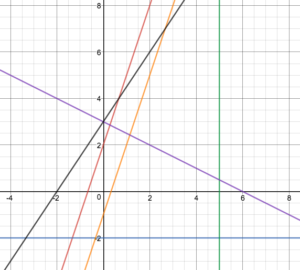(a) $y=3x+2$

(b) $y-4=-\frac{1}{2}(x+2)$

(c) $x=5$

(d) $y=-2$

(e) $3x=y+1$

(f) $2y-3x=6$

## Writing Equations of Lines

### Slope-Intercept Form

Perhaps the most familiar form of a linear equation is slope-intercept form written as $y=mx+b$, where $m=\text{slope}$ and $b=y\text{-intercept}$. Let us begin with the slope.

### The Slope of a Line

The slope of a line refers to the ratio of the vertical change in y over the horizontal change in x between any two points on a line. It indicates the direction in which a line slants as well as its steepness. Slope is sometimes described as rise over run.

$m=\frac{{y}_{2}-{y}_{1}}{{x}_{2}-{x}_{1}}$

If the slope is positive, the line slants upward to the right. If the slope is negative, the line slants downward to the right. As the slope increases, the line becomes steeper. Some examples are shown below. The lines indicate the following slopes: $m=-3$, $m=2$, and $m=\frac{1}{3}$.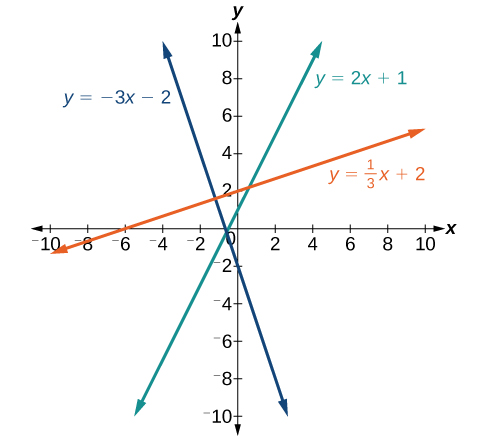### A General Note: The Slope of a Line

The slope of a line, m, represents the change in y over the change in x. Given two points, $\left({x}_{1},{y}_{1}\right)$ and $\left({x}_{2},{y}_{2}\right)$, the following formula determines the slope of a line containing these points:

$m=\frac{{y}_{2}-{y}_{1}}{{x}_{2}-{x}_{1}}$

### Example: Finding the Slope of a Line Given Two Points

Find the slope of a line that passes through the points $\left(2,-1\right)$ and $\left(-5,3\right)$.

### Try It

Find the slope of the line that passes through the points $\left(-2,6\right)$ and $\left(1,4\right)$.

### Example: Identifying the Slope and y-intercept of a Line Given an Equation

Identify the slope and y-intercept given the equation $y=-\frac{3}{4}x - 4$.

### The Point-Slope Form

Given the slope and one point on a line, we can find the equation of the line using point-slope form.

$y-{y}_{1}=m\left(x-{x}_{1}\right)$

This is an important formula, as it will be used in other areas of College Algebra and often in Calculus to find the equation of a tangent line. We need only one point and the slope of the line to use the formula. After substituting the slope and the coordinates of one point into the formula, we simplify it and write it in slope-intercept form.

### A General Note: The Point-Slope Formula

Given one point and the slope, using point-slope form will lead to the equation of a line:

$y-{y}_{1}=m\left(x-{x}_{1}\right)$

### Example: Finding the Equation of a Line Given the Slope and One Point

Write the equation of the line with slope $m=-3$ and passing through the point $\left(4,8\right)$. Write the final equation in slope-intercept form.

### Try It

Given $m=4$, find the equation of the line in slope-intercept form passing through the point $\left(2,5\right)$.

### Example: Finding the Equation of a Line Passing Through Two Given Points

Find the equation of the line passing through the points $\left(3,4\right)$ and $\left(0,-3\right)$. Write the final equation in slope-intercept form.

### Standard Form of a Line

Another way that we can represent the equation of a line is in standard form. Standard form is given as

$Ax+By=C$

where $A$, $B$, and $C$ are integers. The and y-terms are on one side of the equal sign and the constant term is on the other side.

### Example: Finding the Equation of a Line and Writing It in Standard Form

Find the equation of the line with $m=-6$ and passing through the point $\left(\frac{1}{4},-2\right)$. Write the equation in standard form.

### Try It

Find the equation of the line in standard form with slope $m=-\frac{1}{3}$ which passes through the point $\left(1,\frac{1}{3}\right)$.

### Vertical and Horizontal Lines

The equations of vertical and horizontal lines do not require any of the preceding formulas, although we can use the formulas to prove that the equations are correct. The equation of a vertical line is given as

$x=c$

where c is a constant. The slope of a vertical line is undefined, and regardless of the y-value of any point on the line, the x-coordinate of the point will be c.

Suppose that we want to find the equation of a line containing the following points: $\left(-3,-5\right),\left(-3,1\right),\left(-3,3\right)$, and $\left(-3,5\right)$. First, we will find the slope.

$m=\frac{5 - 3}{-3-\left(-3\right)}=\frac{2}{0}$

Zero in the denominator means that the slope is undefined and, therefore, we cannot use point-slope form. However, we can plot the points. Notice that all of the x-coordinates are the same and we find a vertical line through $x=-3$.

The equation of a horizontal line is given as

$y=c$

where c is a constant. The slope of a horizontal line is zero, and for any x-value of a point on the line, the y-coordinate will be c.

Suppose we want to find the equation of a line that contains the following set of points: $\left(-2,-2\right),\left(0,-2\right),\left(3,-2\right)$, and $\left(5,-2\right)$. We can use point-slope form. First, we find the slope using any two points on the line.

$\begin{array}{l}m=\frac{-2-\left(-2\right)}{0-\left(-2\right)}\hfill \\ =\frac{0}{2}\hfill \\ =0\hfill \end{array}$

Use any point for $\left({x}_{1},{y}_{1}\right)$ in the formula, or use the y-intercept.

$\begin{array}{l}y-\left(-2\right)=0\left(x - 3\right)\hfill \\ y+2=0\hfill \\ y=-2\hfill \end{array}$

The graph is a horizontal line through $y=-2$. Notice that all of the y-coordinates are the same.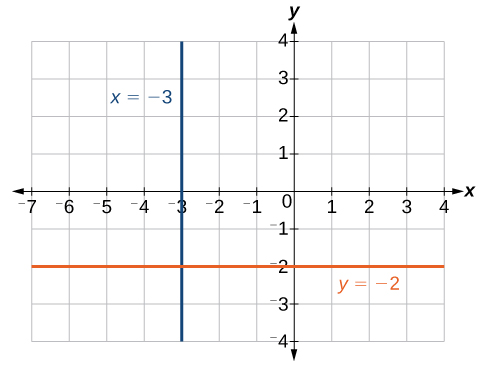The line x = −3 is a vertical line. The line y = −2 is a horizontal line.

### Example: Finding the Equation of a Line Passing Through the Given Points

Find the equation of the line passing through the given points: $\left(1,-3\right)$ and $\left(1,4\right)$.

### Try It

Find the equation of the line passing through $\left(-5,2\right)$ and $\left(2,2\right)$.

## Parallel and Perpendicular Lines

Parallel lines have the same slope and different y-intercepts. Lines that are parallel to each other will never intersect. For example, the figure below shows the graphs of various lines with the same slope, $m=2$.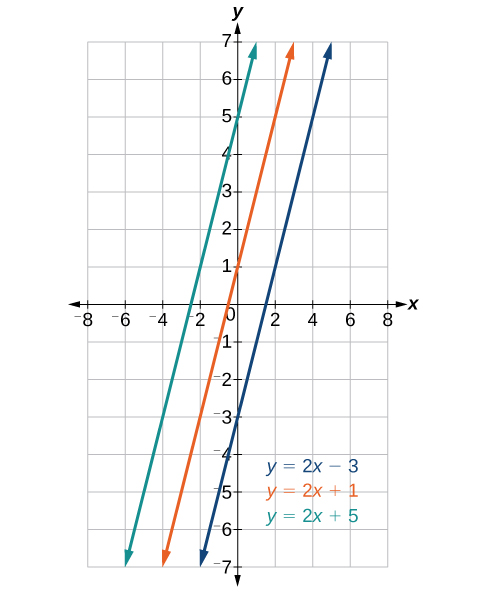Parallel lines have slopes that are the same.

All of the lines shown in the graph are parallel because they have the same slope and different y-intercepts.

Lines that are perpendicular intersect to form a ${90}^{\circ }$ angle. The slope of one line is the negative reciprocal of the other. We can show that two lines are perpendicular if the product of the two slopes is $-1:{m}_{1}\cdot {m}_{2}=-1$. For example, the figure below shows the graph of two perpendicular lines. One line has a slope of 3; the other line has a slope of $-\frac{1}{3}$.

$\begin{array}{l}\text{ }{m}_{1}\cdot {m}_{2}=-1\hfill \\ \text{ }3\cdot \left(-\frac{1}{3}\right)=-1\hfill \end{array}$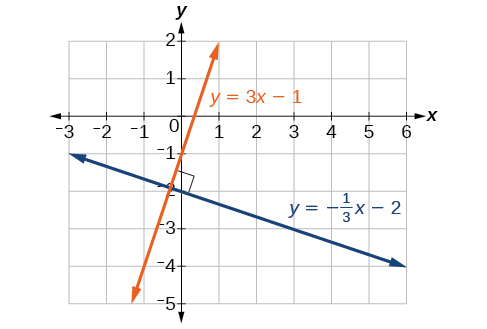Perpendicular lines have slopes that are negative reciprocals of each other.

### Example: Graphing Two Equations and Determining Whether the Lines are Parallel, Perpendicular, or Neither

Graph the equations of the given lines and state whether they are parallel, perpendicular, or neither: $3y=-4x+3$ and $3x - 4y=8$.

### Try It

Graph the two lines and determine whether they are parallel, perpendicular, or neither: $2y-x=10$ and $2y=x+4$.

If we know the equation of a line, we can use what we know about slope to write the equation of a line that is either parallel or perpendicular to the given line.

### Writing Equations of Parallel Lines

Suppose we are given the following equation:

$y=3x+1$

We know that the slope of the line formed by the function is 3. We also know that the y-intercept is (0, 1). Any other line with a slope of 3 will be parallel to $y=3x+1$. So all of the following lines will be parallel to the given line.

$\begin{array}{lll}y=3x+6\hfill & \\ y=3x+1\hfill & \\ y=3x+\frac{2}{3}\hfill \end{array}$

Suppose then we want to write the equation of a line that is parallel to $y=3x+6$ and passes through the point (1, 7). We already know that the slope is 3. We just need to determine which value for b will give the correct line. We can begin with point-slope form of a line and then rewrite it in slope-intercept form.

$\begin{array}{llll}y-{y}_{1}=m\left(x-{x}_{1}\right)\hfill & \\ y - 7=3\left(x - 1\right)\hfill & \\ y - 7=3x - 3\hfill & \\ \text{}y=3x+4\hfill \end{array}$

So $y=3x+4$ is parallel to $y=3x+1$ and passes through the point (1, 7).

### How To: Given the equation of a LINE, write the equation of a line parallel to the given line that passes through A given point

1. Find the slope of the line.
2. Substitute the given values into either point-slope form or slope-intercept form.
3. Simplify.

### Example: Finding a Line Parallel to a Given Line

Find a line parallel to the graph of $y=3x+6$ that passes through the point (3, 0).

### Writing Equations of Perpendicular Lines

We can use a very similar process to write the equation for a line perpendicular to a given line. Instead of using the same slope, however, we use the negative reciprocal of the given slope. Suppose we are given the following line:

$y=2x+4$

The slope of the line is 2 and its negative reciprocal is $-\frac{1}{2}$. Any function with a slope of $-\frac{1}{2}$ will be perpendicular to $y=2x+4$. So all of the following lines will be perpendicular to $y=2x+4$.

$\begin{array}{lll}y=-\frac{1}{2}x+4\hfill & \\ y=-\frac{1}{2}x+2\hfill & \\ y=-\frac{1}{2}x-\frac{1}{2}\hfill \end{array}$

As before, we can narrow down our choices for a particular perpendicular line if we know that it passes through a given point. Suppose then we want to write the equation of a line that is perpendicular to $y=2x+4$ and passes through the point (4, 0). We already know that the slope is $-\frac{1}{2}$. Now we can use the point to find the y-intercept by substituting the given values into slope-intercept form and solving for b.

$\begin{array}{lllll}y=mx+b\hfill & \\ 0=-\frac{1}{2}\left(4\right)+b\hfill & \\ 0=-2+b\hfill \\ 2=b\hfill & \\ b=2\hfill \end{array}$

The equation for the function with a slope of $-\frac{1}{2}$ and a y-intercept of 2 is $y=-\frac{1}{2}x+2$.

So $y=-\frac{1}{2}x+2$ is perpendicular to $y=2x+4$ and passes through the point (4, 0). Be aware that perpendicular lines may not look obviously perpendicular on a graphing calculator unless we use the square zoom feature.

### Q & A

A horizontal line has a slope of zero and a vertical line has an undefined slope. These two lines are perpendicular, but the product of their slopes is not –1. Doesn’t this fact contradict the definition of perpendicular lines?

No. For two perpendicular linear functions, the product of their slopes is –1. As you will learn later, a vertical line is not a function so the definition is not contradicted.

### How To: Given the equation of a LINE, write the equation of a line Perpendicular to the given line that passes through A given point

1. Find the slope of the given line.
2. Determine the negative reciprocal of the slope.
3. Substitute the slope and point into either point-slope form or slope-intercept form.
4. Simplify.

### Example: Finding the Equation of a Perpendicular Line

Find the equation of a line perpendicular to $y=3x+3$ that passes through the point (3, 0).

### Try It

Given the line $y=2x - 4$, write an equation for the line passing through (0, 0) that is

1. parallel to y
2. perpendicular to y

### How To: Given two points on a line, write the equation of A perpendicular line that passes through A Third point

1. Determine the slope of the line passing through the points.
2. Find the negative reciprocal of the slope.
3. Substitute the slope and point into either point-slope form or slope-intercept form.
4. Simplify.

### Example: Finding the Equation of a Perpendicular Line

A line passes through the points (–2, 6) and (4, 5). Find the equation of a perpendicular line that passes through the point (4, 5).

### Try It

A line passes through the points (–2, –15) and (2, –3). Find the equation of a perpendicular line that passes through the point (6, 4).

### Writing the Equations of Lines Parallel or Perpendicular to a Given Line

As we have learned, determining whether two lines are parallel or perpendicular is a matter of finding the slopes. To write the equation of a line parallel or perpendicular to another line, we follow the same principles as we do for finding the equation of any line. After finding the slope, use point-slope form to write the equation of the new line.

### Example: Writing the Equation of a Line Parallel to a Given Line

Write the equation of line parallel to a $5x+3y=1$ which passes through the point $\left(3,5\right)$.

### Try It

Find the equation of the line parallel to $5x=7+y$ which passes through the point $\left(-1,-2\right)$.

### Example: Finding the Equation of a Perpendicular Line

Find the equation of the line perpendicular to $5x - 3y+4=0$ which goes through the point $\left(-4,1\right)$.

## Key Concepts

• Given two points, we can find the slope of a line using the slope formula.
• We can identify the slope and y-intercept of an equation in slope-intercept form.
• We can find the equation of a line given the slope and a point.
• We can also find the equation of a line given two points. Find the slope and use point-slope form.
• The standard form of a line has no fractions.
• Horizontal lines have a slope of zero and are defined as $y=c$, where c is a constant.
• Vertical lines have an undefined slope (zero in the denominator) and are defined as $x=c$, where c is a constant.
• Parallel lines have the same slope and different y-intercepts.
• Perpendicular lines have slopes that are negative reciprocals of each other unless one is horizontal and the other is vertical.

## Glossary

slope
the change in y-values over the change in x-values